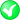#Boost C++ Libraries

...one of the most highly regarded and expertly designed C++ library projects in the world.

##### correct (with strategy)

Corrects a geometry.

###### Description

Corrects a geometry: all rings which are wrongly oriented with respect to their expected orientation are reversed. To all rings which do not have a closing point and are typed as they should have one, the first point is appended. Also boxes can be corrected.

###### Synopsis

```template<typename Geometry, typename Strategy>
void correct(Geometry & geometry, Strategy const & strategy)```

###### Parameters

Type

Concept

Name

Description

Geometry &

Any type fulfilling a Geometry Concept

geometry

A model of the specified concept which will be corrected if necessary

Strategy const &

Any type fulfilling a Area Strategy Concept

strategy

The strategy which will be used for area calculations

Either

`#include <boost/geometry.hpp>`

Or

`#include <boost/geometry/algorithms/correct.hpp>`

###### Conformance

The function correct is not defined by OGC.

###### Supported geometries

Geometry

Status

PointSegmentBoxLinestringRingPolygonMultiPointMultiLinestringMultiPolygonVariant###### Behavior

Case

Behavior

Ring

Ring is corrected

Polygon

Polygon is corrected

Multi Polygon

Multi Polygon is corrected

Box

Box is corrected with respect to minimal and maximal corners

Other geometries

Nothing happens, geometry is unchanged

Linear

###### Example

Shows how to correct a polygon with respect to its orientation and closure

```#include <iostream>

#include <boost/geometry.hpp>
#include <boost/geometry/geometries/polygon.hpp>

BOOST_GEOMETRY_REGISTER_BOOST_TUPLE_CS(cs::cartesian)

#include <boost/assign.hpp>

int main()
{
using boost::assign::tuple_list_of;

typedef boost::geometry::model::polygon
<
boost::tuple<int, int>
> clockwise_closed_polygon;

clockwise_closed_polygon cwcp;

// Fill it counterclockwise (so wrongly), forgetting the closing point
boost::geometry::exterior_ring(cwcp) = tuple_list_of(0, 0)(10, 10)(0, 9);

// Add a counterclockwise closed inner ring (this is correct)
boost::geometry::interior_rings(cwcp).push_back(tuple_list_of(1, 2)(4, 6)(2, 8)(1, 2));

// Its area should be negative (because of wrong orientation)
//     and wrong (because of omitted closing point)
double area_before = boost::geometry::area(cwcp);

// Correct it!
boost::geometry::correct(cwcp);

// Check its new area
double area_after = boost::geometry::area(cwcp);

// And output it
std::cout << boost::geometry::dsv(cwcp) << std::endl;
std::cout << area_before << " -> " << area_after << std::endl;

return 0;
}
```

Output:

```(((0, 0), (0, 9), (10, 10), (0, 0)), ((1, 2), (4, 6), (2, 8), (1, 2)))
-7 -> 38
```